# Generics Array Lists and arrays n A Array

• Slides: 16
Download presentation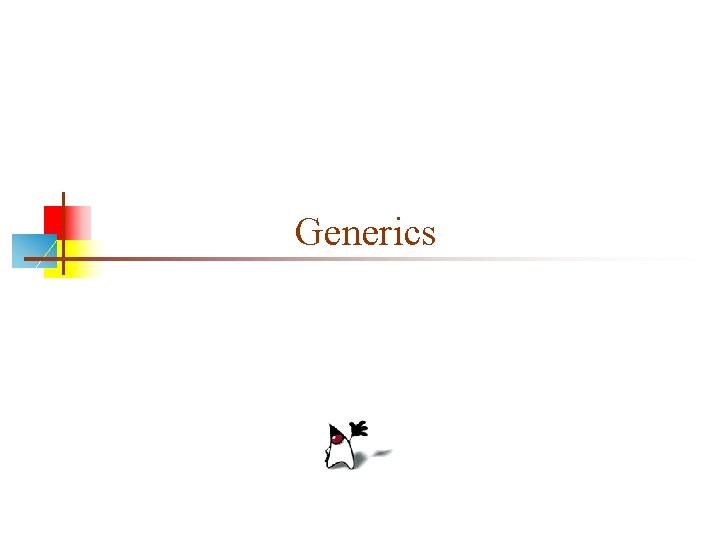GenericsArray. Lists and arrays n A Array. List is like an array of Objects, but. . . n n To create an Array. List: n n n Arrays use [ ] syntax; Array. Lists use object syntax An Array. List expands as you add things to it Arrays can hold primitives or objects, but Array. Lists can only hold objects Array. List my. List = new Array. List(); Or, since an Array. List is a kind of List, List my. List = new Array. List(); To use an Array. List, n boolean add(Object obj) n Object set(int index, Object obj) n Object get(int index)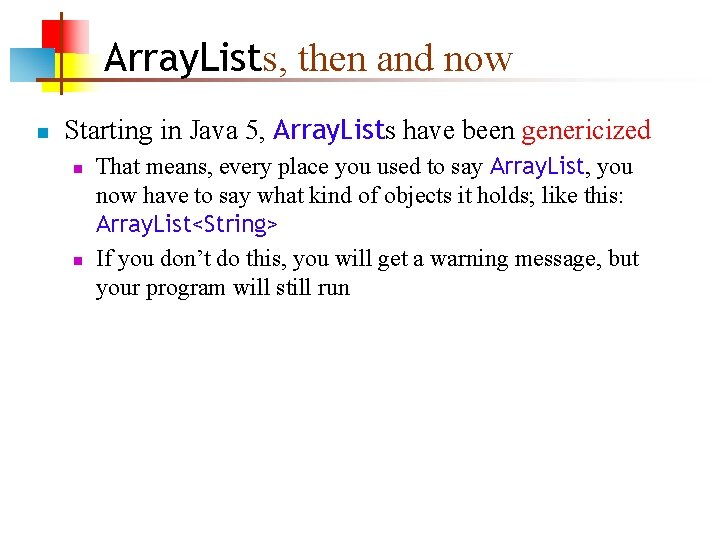Array. Lists, then and now n Starting in Java 5, Array. Lists have been genericized n n That means, every place you used to say Array. List, you now have to say what kind of objects it holds; like this: Array. List<String> If you don’t do this, you will get a warning message, but your program will still run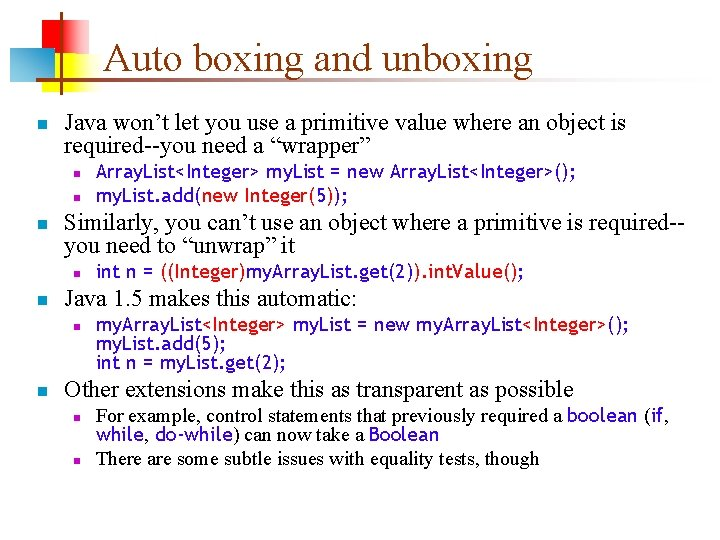Auto boxing and unboxing n Java won’t let you use a primitive value where an object is required--you need a “wrapper” n n n Similarly, you can’t use an object where a primitive is required-you need to “unwrap” it n n int n = ((Integer)my. Array. List. get(2)). int. Value(); Java 1. 5 makes this automatic: n n Array. List<Integer> my. List = new Array. List<Integer>(); my. List. add(new Integer(5)); my. Array. List<Integer> my. List = new my. Array. List<Integer>(); my. List. add(5); int n = my. List. get(2); Other extensions make this as transparent as possible n n For example, control statements that previously required a boolean (if, while, do-while) can now take a Boolean There are some subtle issues with equality tests, though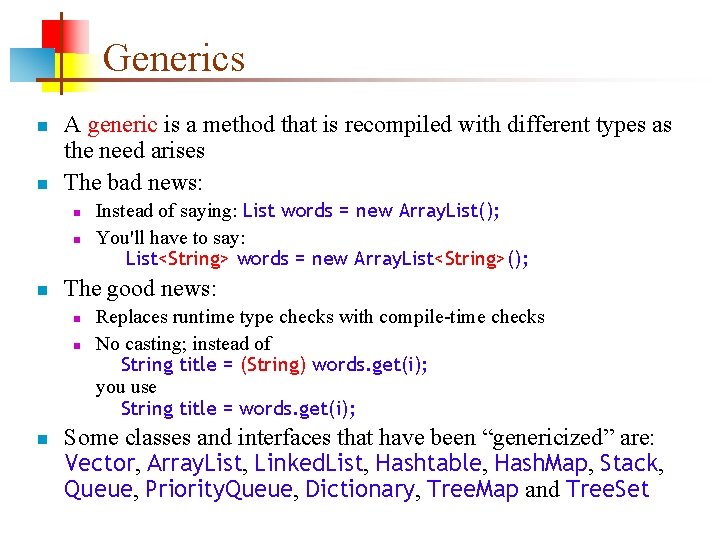Generics n n A generic is a method that is recompiled with different types as the need arises The bad news: n n n The good news: n n n Instead of saying: List words = new Array. List(); You'll have to say: List<String> words = new Array. List<String>(); Replaces runtime type checks with compile-time checks No casting; instead of String title = (String) words. get(i); you use String title = words. get(i); Some classes and interfaces that have been “genericized” are: Vector, Array. List, Linked. List, Hashtable, Hash. Map, Stack, Queue, Priority. Queue, Dictionary, Tree. Map and Tree. SetGeneric Iterators n An Iterator is an object that will let you step through the elements of a list one at a time n n Iterators have also been genericized: n n List<String> list. Of. Strings = new Array. List<String>(); . . . for (Iterator i = list. Of. Strings. iterator(); i. has. Next(); ) { String s = (String) i. next(); System. out. println(s); } List<String> list. Of. Strings = new Array. List<String>(); . . . for (Iterator<String> i = list. Of. Strings. iterator(); i. has. Next(); ) { String s = i. next(); System. out. println(s); } You can also use the new for statement (to be discussed)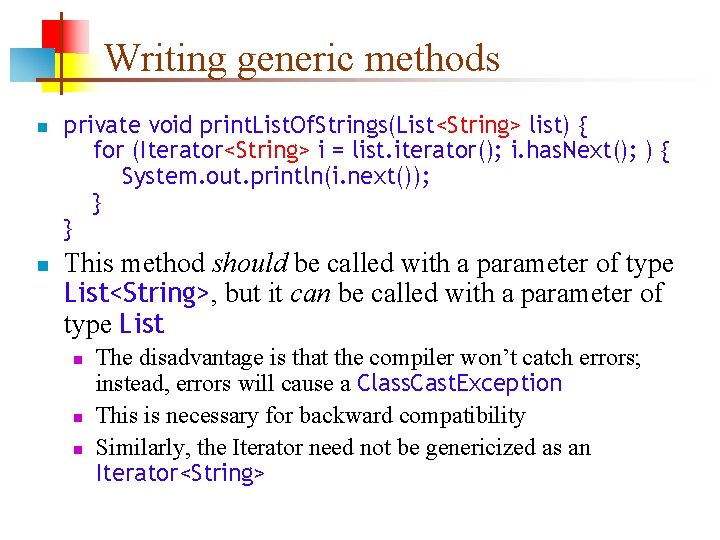Writing generic methods n n private void print. List. Of. Strings(List<String> list) { for (Iterator<String> i = list. iterator(); i. has. Next(); ) { System. out. println(i. next()); } } This method should be called with a parameter of type List<String>, but it can be called with a parameter of type List n n n The disadvantage is that the compiler won’t catch errors; instead, errors will cause a Class. Cast. Exception This is necessary for backward compatibility Similarly, the Iterator need not be genericized as an Iterator<String>Type wildcards n Here’s a simple (no generics) method to print out any list: n n The above still works in Java 1. 5, but now it generates warning messages n n private void print. List(List list) { for (Iterator i = list. iterator(); i. has. Next(); ) { System. out. println(i. next()); } } Java 1. 5 incorporates lint (like C lint) to look for possible problems You should eliminate all errors and warnings in your final code, so you need to tell Java that any type is acceptable: n private void print. List. Of. Strings(List<? > list) { for (Iterator<? > i = list. iterator(); i. has. Next(); ) { System. out. println(i. next()); } }Writing your own generic types n public class Box<T> { private List<T> contents; public Box() { contents = new Array. List<T>(); } public void add(T thing) { contents. add(thing); } public T grab() { if (contents. size() > 0) return contents. remove(0); else return null; n n } Sun’s recommendation is to use single capital letters (such as T) for types If you have more than a couple generic types, though, you should use better namesNew for statement n n n The syntax of the new statement is for(type var : array) {. . . } or for(type var : collection) {. . . } Example: for(float x : my. Real. Array) { my. Real. Sum += x; } For a collection class that has an Iterator, instead of for (Iterator iter = c. iterator(); iter. has. Next(); ) ((Timer. Task) iter. next()). cancel(); you can now say for (Timer. Task task : c) task. cancel();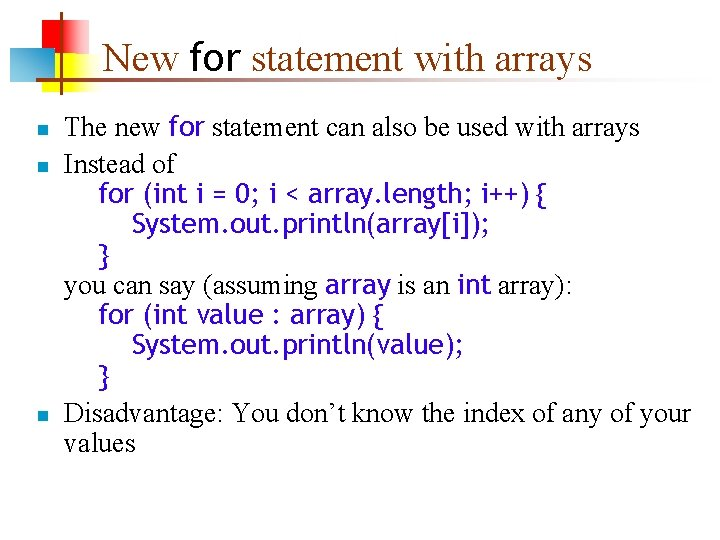New for statement with arrays n n n The new for statement can also be used with arrays Instead of for (int i = 0; i < array. length; i++) { System. out. println(array[i]); } you can say (assuming array is an int array): for (int value : array) { System. out. println(value); } Disadvantage: You don’t know the index of any of your values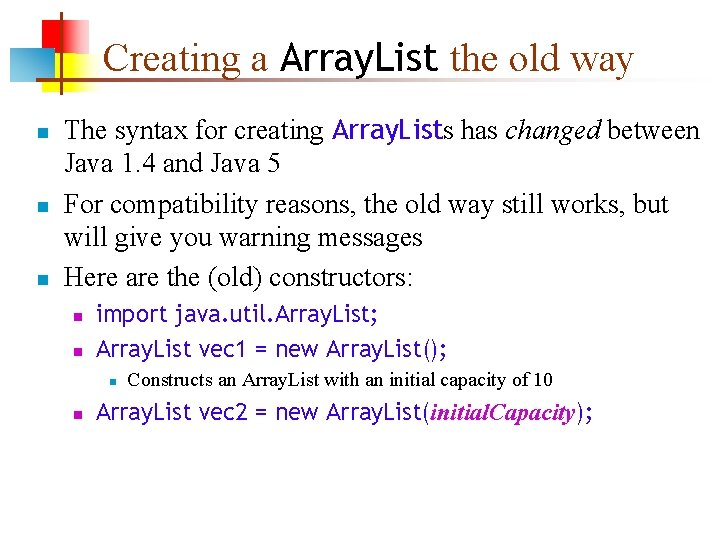Creating a Array. List the old way n n n The syntax for creating Array. Lists has changed between Java 1. 4 and Java 5 For compatibility reasons, the old way still works, but will give you warning messages Here are the (old) constructors: n n import java. util. Array. List; Array. List vec 1 = new Array. List(); n n Constructs an Array. List with an initial capacity of 10 Array. List vec 2 = new Array. List(initial. Capacity);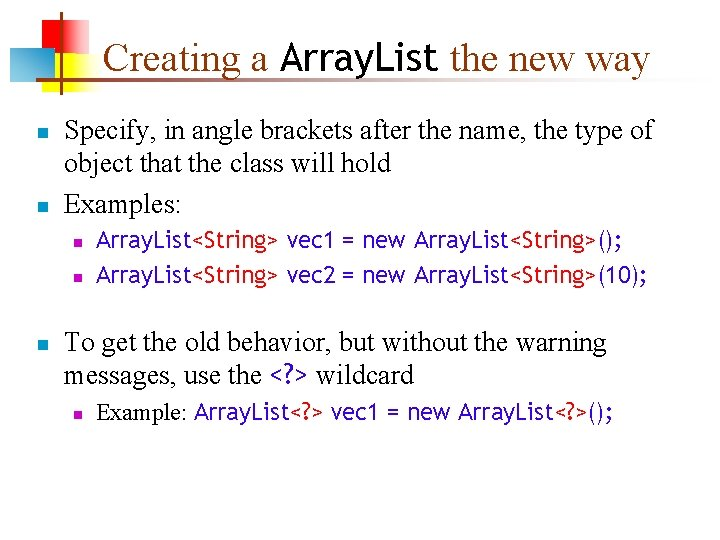Creating a Array. List the new way n n Specify, in angle brackets after the name, the type of object that the class will hold Examples: n n n Array. List<String> vec 1 = new Array. List<String>(); Array. List<String> vec 2 = new Array. List<String>(10); To get the old behavior, but without the warning messages, use the <? > wildcard n Example: Array. List<? > vec 1 = new Array. List<? >();Accessing with and without generics n Object get(int index) n n Using get the old way: n n Array. List my. List = new Array. List(); my. List. add("Some string"); String s = (String)my. List. get(0); Using get the new way: n n Returns the component at position index Array. List<String> my. List = new Array. List<String>(); my. List. add("Some string"); String s = my. List. get(0); Notice that casting is no longer necessary when we retrieve an element from a “genericized” Array. List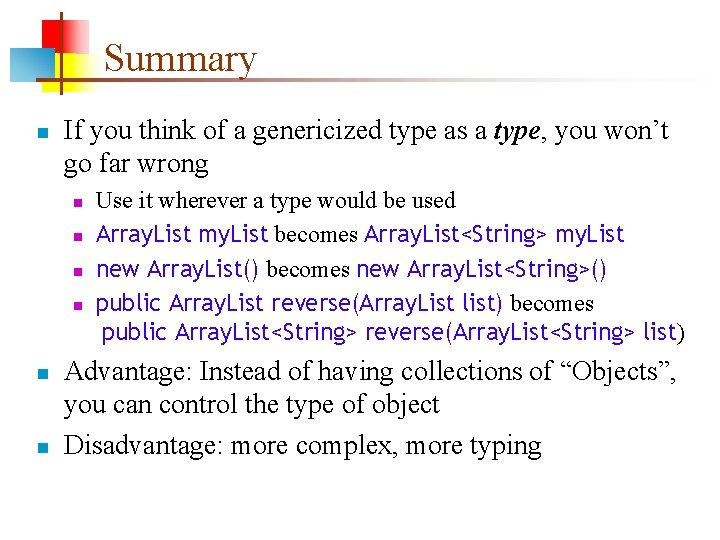Summary n If you think of a genericized type as a type, you won’t go far wrong n n n Use it wherever a type would be used Array. List my. List becomes Array. List<String> my. List new Array. List() becomes new Array. List<String>() public Array. List reverse(Array. List list) becomes public Array. List<String> reverse(Array. List<String> list) Advantage: Instead of having collections of “Objects”, you can control the type of object Disadvantage: more complex, more typingThe End